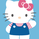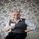# ------ Chop + Vdub FX SniperVX3-- for day trading -- use 1-5 min

‎3648‎ مشاهدة
3648
Hello everyone,

I've adapted two scripts chop + vdub for day trading use. Use 1-2-3-4-5 minutes for test.
Back test for this Week only!!! Change the timestamps for using in the next week etc!!
```//@version=2
//╭╮╱╱╭╮╭╮╱╱╭╮
//┃╰╮╭╯┃┃┃╱╱┃┃
//╰╮┃┃╭┻╯┣╮╭┫╰━┳╮╭┳━━╮
//╱┃╰╯┃╭╮┃┃┃┃╭╮┃┃┃┃━━┫
//╱╰╮╭┫╰╯┃╰╯┃╰╯┃╰╯┣━━┃  ///extended vDr
//╱╱╰╯╰━━┻━━┻━━┻━━┻━━╯
//╭━━━┳╮╱╱╱╱╱╱╱╭╮
//┃╭━╮┃┃╱╱╱╱╱╱╱┃┃
//┃┃╱╰┫╰━┳━━┳━╮╭━╮╭━━┫┃
//┃┃╱╭┫╭╮┃╭╮┃╭╮┫╭╮┫┃━┫┃
//┃╰━╯┃┃┃┃╭╮┃┃┃┃┃┃┃┃━┫╰╮
//╰━━━┻╯╰┻╯╰┻╯╰┻╯╰┻━━┻━╯
//━╯
// http://www.vdubus.co.uk/
strategy(title='------ Chop + Vdub FX SniperVX3', shorttitle='----Chop+Vdub_FX_SniperVX3', overlay=true, pyramiding=1, initial_capital=1000, currency=currency.USD)

//Candle body resistance Channel-----------------------------//
len = 34
src = input(close, title="Candle body resistance Channel")
out = sma(src, len)
last8h = highest(close, 13)
lastl8 = lowest(close, 13)
bearish = cross(close,out) == 1 and falling(close, 1)
bullish = cross(close,out) == 1 and rising(close, 1)
channel2=input(false, title="Bar Channel On/Off")
ul2=plot(channel2?last8h:last8h==nz(last8h)?last8h:na, color=black, linewidth=1, style=linebr, title="Candle body resistance level top", offset=0)
ll2=plot(channel2?lastl8:lastl8==nz(lastl8)?lastl8:na, color=black, linewidth=1, style=linebr, title="Candle body resistance level bottom", offset=0)
//fill(ul2, ll2, color=black, transp=95, title="Candle body resistance Channel")

//-----------------Support and Resistance
RST = input(title='Support / Resistance length:', type=integer, defval=10)
RSTT = valuewhen(high >= highest(high, RST), high, 0)
RSTB = valuewhen(low <= lowest(low, RST), low, 0)
RT2 = plot(RSTT, color=RSTT != RSTT ? na : red, linewidth=1, offset=+0)
RB2 = plot(RSTB, color=RSTB != RSTB ? na : green, linewidth=1, offset=0)

//--------------------Trend colour ema------------------------------------------------//
src0 = close, len0 = input(13, minval=1, title="EMA 1")
ema0 = ema(src0, len0)
direction = rising(ema0, 2) ? +1 : falling(ema0, 2) ? -1 : 0
plot_color = direction > 0  ? lime: direction < 0 ? red : na
plot(ema0, title="EMA", style=line, linewidth=1, color = plot_color)

//-------------------- ema 2------------------------------------------------//
src02 = close, len02 = input(21, minval=1, title="EMA 2")
ema02 = ema(src02, len02)
direction2 = rising(ema02, 2) ? +1 : falling(ema02, 2) ? -1 : 0
plot_color2 = direction2 > 0  ? lime: direction2 < 0 ? red : na
plot(ema02, title="EMA Signal 2", style=line, linewidth=1, color = plot_color2)

//=============Hull MA//
show_hma = input(false, title="Display Hull MA Set:")
hma_src = input(close, title="Hull MA's Source:")
hma_base_length = input(8, minval=1, title="Hull MA's Base Length:")
hma_length_scalar = input(5, minval=0, title="Hull MA's Length Scalar:")
hullma(src, length)=>wma(2*wma(src, length/2)-wma(src, length), round(sqrt(length)))
plot(not show_hma ? na : hullma(hma_src, hma_base_length+hma_length_scalar*6), color=black, linewidth=2, title="Hull MA")

rangeup = close+tr
rangedown = close-tr
over() => high > rangeup and close > open
under() => low < rangedown and close < open

//============ signal Generator ==================================//
Piriod=input('720')
ch1 = security(tickerid, Piriod, open)
ch2 = security(tickerid, Piriod, close)

longCondition = crossover(security(tickerid, Piriod, close),security(tickerid, Piriod, open))
//if (longCondition )
//    strategy.entry("Vdub Long", strategy.long, when = over())

shortCondition = crossunder(security(tickerid, Piriod, close),security(tickerid, Piriod, open))
//if (shortCondition)
//    strategy.entry("Vdub Short", strategy.short, when = under())
//if(over())
//    strategy.exit("Vdub Short")
//if(under())
//    strategy.exit("Vdub Long")
//strategy.exit("Vdub Short", loss = 10)
//strategy.exit("Vdub Long", loss = 10)

strategy.entry("CBL", strategy.long, when = over() and time >= (timestamp(2016, 03, 07, 09, 30)) and time < (timestamp(2016, 03, 12, 00, 00)))
strategy.entry("CBS", strategy.short, when = under() and time >= (timestamp(2016, 03, 07, 09, 30)) and time < (timestamp(2016, 03, 12, 00, 00)))

strategy.exit(id="XVdubL",from_entry="CBL", when = under() or shortCondition, loss=20)
strategy.exit(id="XVdubS",from_entry="CBS", when = over() or longCondition, loss=20)

///////////////////////////////////////////////////////////////////////////////////////////```I don't understand why so many people on this site are creating strategies based on future data. Everyday I contemplate on leaving this site. I've wasted countless days on backtests that rely on future data. This is the only website I know that allows this to happen. Unreal
ردCosmonautCalls
@CosmonautCalls, with version2, security function has lookahead enabled by default, with version3 this is disabled by default. Basically longCondition/shortCondition are using the future candle close price for backtest, so they are using 1 leading candle in this case, and resulting in wrong backtests
ردThis strategy rely on future data (repaints) and can not be used in real life. Please vote up for fixing this issue: https://getsatisfaction.com/tradingview/topics/strategies-and-indicators-are-repainting
رد